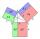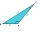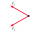# Triangle + The Law of Cosines - math problems

#### Number of problems found: 36

• LawsFrom which law follows directly the validity of Pythagoras' theorem in the right triangle? ?
• Scalene triangleSolve the triangle: A = 50°, b = 13, c = 6
• Triangle ABCTriangle ABC has side lengths m-1, m-2, m-3. What has to be m to be triangle a) rectangular b) acute-angled?
• Angles by cosine lawCalculate the size of the angles of the triangle ABC, if it is given by: a = 3 cm; b = 5 cm; c = 7 cm (use the sine and cosine theorem).
• Greatest angleCalculate the greatest triangle angle with sides 197, 208, 299.
• Largest angle of the triangleCalculate the largest angle of the triangle whose sides have the sizes: 2a, 3/2a, 3a
• Find the areaFind the area of the triangle with the given measurements. Round the solution to the nearest hundredth if necessary. A = 50°, b = 30 ft, c = 14 ft
• Heron backlawCalculate missing side in a triangle with sides 17 and 34 and area 275.
• Medians of isosceles triangleThe isosceles triangle has a base ABC |AB| = 16 cm and 10 cm long arm. What are the length of medians?
• Triangle from medianCalculate the perimeter, content, and magnitudes of the remaining angles of triangle ABC, given: a = 8.4; β = 105° 35 '; and median ta = 12.5.
• Side cIn △ABC a=2, b=4 and ∠C=100°. Calculate length of the side c.
• Triangle and its heightsCalculate the length of the sides of the triangle ABC, if va=5 cm, vb=7 cm and side b is 5 cm shorter than side a.
• ABCDAC= 40cm , angle DAB=38 , angle DCB=58 , angle DBC=90 , DB is perpendicular on AC , find BD and AD
• Diagonals in diamondIn the rhombus is given a = 160 cm, alpha = 60 degrees. Calculate the length of the diagonals.
• DiagonalsCalculate the length of the diagonals of the rhombus if its side is long 5 and one of its internal angle is 80°.
• Calculate 2Calculate the largest angle of the triangle whose side are 5.2cm, 3.6cm, and 2.1cm
• The angle of viewDetermine the angle of view at which the observer sees a rod 16 m long when it is 18 m from one end and 27 m from the other.
• Triangle SASCalculate the triangle area and perimeter, if the two sides are 51 cm and 110 cm long and angle them clamped is 130 °.
• TrianglePlane coordinates of vertices: K[11, -10] L[10, 12] M[1, 3] give Triangle KLM. Calculate its area and its interior angles.
• Mass pointTwo equal forces of 30 Newtons act on a mass point. Find the magnitude of the resultant force if these forces form an angle of 42°.

Do you have an interesting mathematical word problem that you can't solve it? Submit a math problem, and we can try to solve it.

We will send a solution to your e-mail address. Solved examples are also published here. Please enter the e-mail correctly and check whether you don't have a full mailbox.

Please do not submit problems from current active competitions such as Mathematical Olympiad, correspondence seminars etc...

Cosine rule uses trigonometric SAS triangle calculator. See also our trigonometric triangle calculator. Triangle Problems. The Law of Cosines - math word problems.